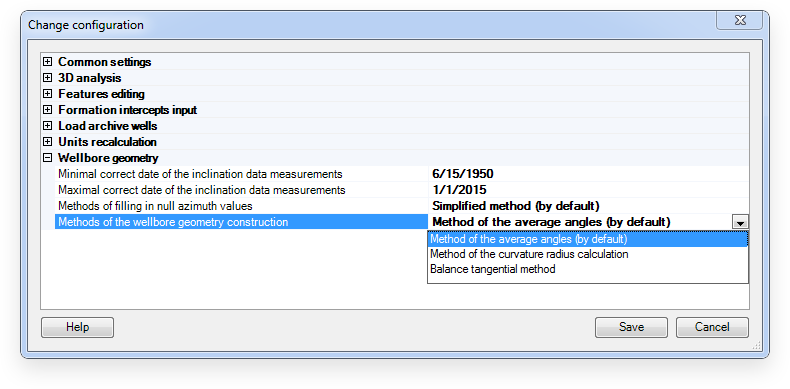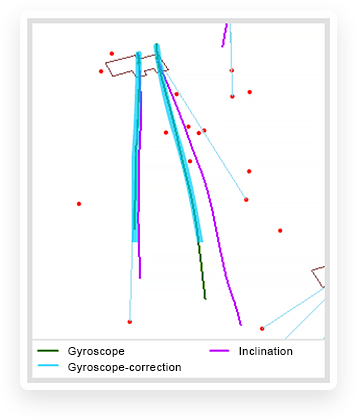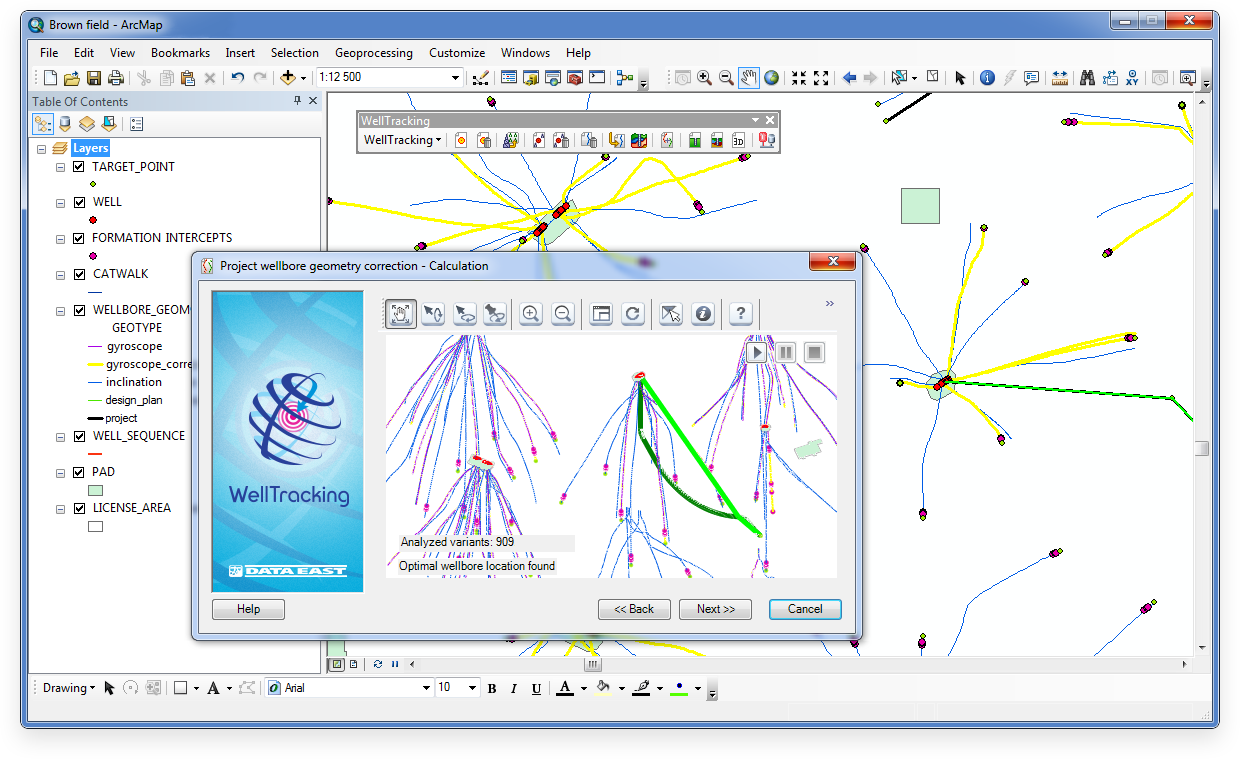### Calculating wellbore geometry

Wellbore geometry can be calculated using:

• Method of the curvature radius calculation
• Method of the average angles
• Balance tangential method### Calculating corrected gyroscope

Calculation of wellbore tracks using the gyroscope and deviation approximation method.